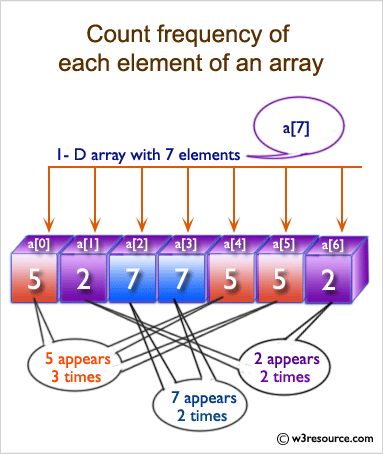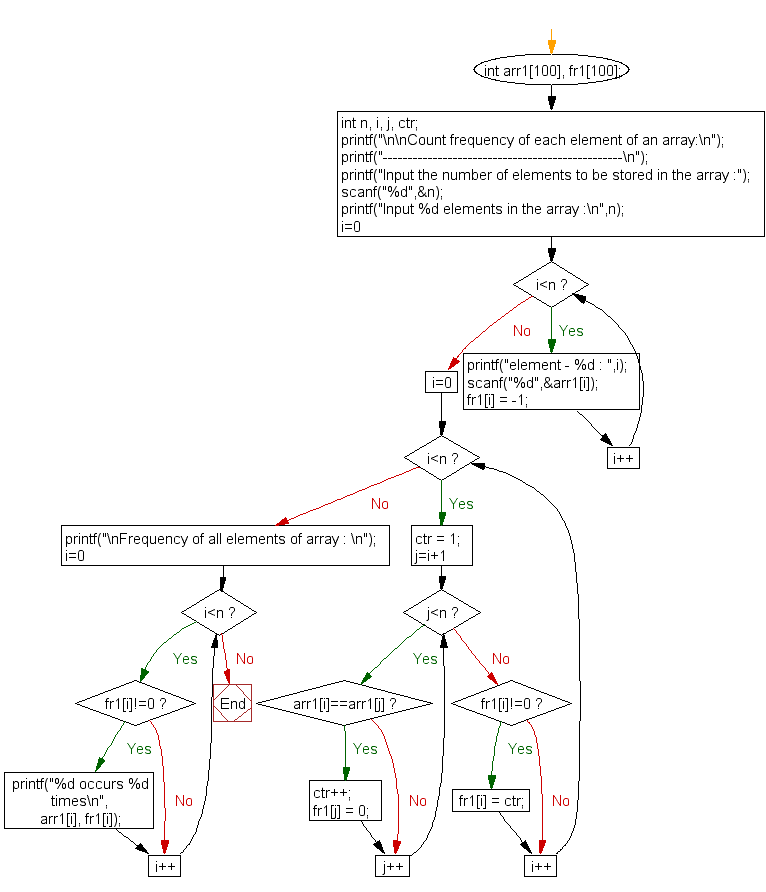﻿ C exercises: Count the frequency of each element of an array - w3resource# C Exercises: Count the frequency of each element of an array

## C Array: Exercise-8 with Solution

Write a program in C to count the frequency of each element of an array.

Pictorial Presentation:Sample Solution:

C Code:

``````#include <stdio.h>

void main()
{
int arr1, fr1;
int n, i, j, ctr;

printf("\n\nCount frequency of each element of an array:\n");
printf("------------------------------------------------\n");

printf("Input the number of elements to be stored in the array :");
scanf("%d",&n);

printf("Input %d elements in the array :\n",n);
for(i=0;i<n;i++)
{
printf("element - %d : ",i);
scanf("%d",&arr1[i]);
fr1[i] = -1;
}
for(i=0; i<n; i++)
{
ctr = 1;
for(j=i+1; j<n; j++)
{
if(arr1[i]==arr1[j])
{
ctr++;
fr1[j] = 0;
}
}

if(fr1[i]!=0)
{
fr1[i] = ctr;
}
}
printf("\nThe frequency of all elements of array : \n");
for(i=0; i<n; i++)
{
if(fr1[i]!=0)
{
printf("%d occurs %d times\n", arr1[i], fr1[i]);
}
}
}
```
```

Sample Output:

```Count frequency of each element of an array:
------------------------------------------------
Input the number of elements to be stored in the array :3
Input 3 elements in the array :
element - 0 : 25
element - 1 : 12
element - 2 : 43

The frequency of all elements of array :
25 occurs 1 times
12 occurs 1 times
43 occurs 1 times
```

Flowchart:C Programming Code Editor:

Improve this sample solution and post your code through Disqus.

What is the difficulty level of this exercise?

﻿